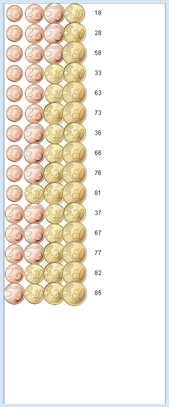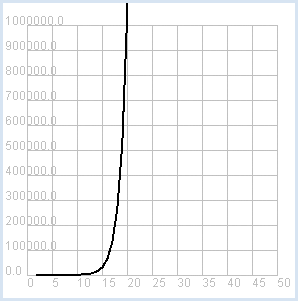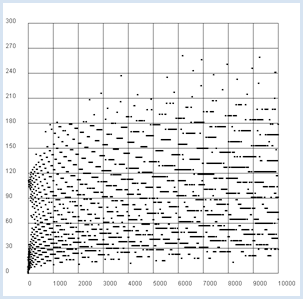deutsch     english    français     Print

## 10.2 UNSOLVABLE PROBLEMS### INTRODUCTION

Increasingly many problems can be solved with clever computer programs. In this chapter, however, you will be confronted with questions that can be simply formulated but that may never be algorithmically solvable, despite the rapid evolution of computers and enormous scientific effort.

 PROGRAMMING CONCEPTS: Unsolvable problems, subset sum problem, enumeration methods, combinatorial explosion, polynomial order, undecidable problem

### UNSOLVABLE PROBLEMS

There are still some problems that are unsolved, even though they are easy to formulate and especially important in practice. One of these, known as the subset sum problem, can be described as follows [more...The partial sum problem is a special case of the knapsack problem
and how this also NP-complete
]:

 You have a number of coins in your purse and you must pay a certain amount with it (without getting change back). Is it possible with the existing coins, and if so, what coins should you pay with? In your first program you will first learn to handle the coins. You save the names of the Euro coins with the values 1, 2, 5, 10, 20, 50 cents in the list coins. The function value() returns the value of a coin. The purse is modeled as a list (or a tuple) named moneybag and contains the names of the coins contained in the purse. The function getSum(moneybag) then returns the total value of all coins in the purse. The purse should initially contain exactly one of each coin. You create all possible variations of coin combinations with 1, 2, 3, 4, 5 or 6 coins and display them in a JGameGrid window. To do this, you make an actor out of each coin of moneybag in showMoneybag(moneybag, y) and display them in the game window in the line y.```from gamegrid import *
import itertools

coins = ["one", "two", "five", "ten", "twenty", "fifty"]

def value(coin):
if coin == "one":
return 1
if coin == "two":
return 2
if coin == "five":
return 5
if coin == "ten":
return 10
if coin == "twenty":
return 20
if coin == "fifty":
return 50
return 0

def getSum(moneybag):
count = 0
for coin in moneybag:
count += value(coin)
return count

def showMoneybag(moneybag, y):
x = 0
for coin in moneybag:
loc = Location(x, y)
removeActor(getOneActorAt(loc))
coinActor = Actor("sprites/" + coin + "cent.png")
x += 1

makeGameGrid(8, 20, 40, False)
setBgColor(Color.white)
show()

n = 6
k = 1
while k <= n:
combinations = list(itertools.combinations(coins, k))
setTitle("(n, k) = (" + str(n) + ", " + str(k) + ") nb = "
+ str(len(combinations)))
y = 0
for moneybag in combinations:
showMoneybag(moneybag, y)
y += 1
getKeyCodeWait()
removeAllActors()
k += 1

```
Highlight program code (Ctrl+C copy, Ctrl+V paste)

### MEMO

 The combinations of k elements that you can build from a list of n, are easily retrievable with the function combinations() from the module itertools. You have to convert the return value to a list, from which the found combinations can then be retrieved (as tuples). As you can see, the obtained combinations are ordered in a way similar to how you would reasonably order them by hand. You can calculate the number of combinations of n elements to the order k as follows, as you know:where n! is the factorial, meaning the product of all numbers from 1 to n. In our case n = 6 can result in 6, 15, 20, 15, 6, 1, so a total of 63 combinations.

 You can now solve the subset sum problem of the purse as follows: Determine all combinations of the existing coins and examine them individually to see if their sum adds up to the desired value. This enumeration method is probably not the best to use, but it is definitely correct and it provides all possible solutions. For a purse that contains 3 one-cent, 1 two-cents, 2 five-cents, 4 ten-cents, 2 twenty-cents, and 3 fifty-cents coins (15 coins in total) it would already become difficult to find the solution by hand. You only write all different sets of coins that amount to 1 Euro.```from gamegrid import *
import itertools

coins = ["one", "one", "one", "two", "five", "five",
"ten", "ten", "ten", "ten", "twenty", "twenty",
"fifty", "fifty", "fifty"]

def value(coin):
if coin == "one":
return 1
if coin == "two":
return 2
if coin == "five":
return 5
if coin == "ten":
return 10
if coin == "twenty":
return 20
if coin == "fifty":
return 50
return 0

def getSum(moneybag):
count = 0
for coin in moneybag:
count += value(coin)
return count

def showMoneybag(moneybag, y):
x = 0
for coin in moneybag:
loc = Location(x, y)
removeActor(getOneActorAt(loc))
coinActor = Actor("sprites/" + coin + "cent.png")
x += 1

makeGameGrid(15, 20, 40, False)
setBgColor(Color.white)
show()

target = 100

k = 1
result = []
count = 0
while k <= len(coins):
combinations = tuple(itertools.combinations(coins, k))
nb = len(combinations)
for moneybag in combinations:
count += 1
count = getSum(moneybag)
if count == target:
if not moneybag in result:
result.append(moneybag)
k += 1

y = 0
for moneybag in result:
showMoneybag(moneybag, y)
y += 1
setTitle("Step: " + str(count) + ". number of solutions for the count  "
+ str(target) + ": " + str(len(result)))
```
Highlight program code (Ctrl+C copy, Ctrl+V paste)

### MEMO

 With only 15 coins there are already 32,767 steps necessary in order to solve the subset sum problem using the enumeration method. Your enjoyment of solving problems with the computer is unfortunately spoiled once you try with a slightly larger purse of money, let's say 50 or 100 coins. If you count the necessary steps it takes for a purse with n coins and display them in a graph, there is a downright combinatorial explosion at n = 20 and you reach the limit of what is possible [more... Other limits you see in the book "Hromkovic, Seven Wonders of the computer science"].```from gpanel import *
from math import factorial

z = 100

def nbCombi(n, k):
return factorial(n) / factorial(k) / factorial(n - k)

makeGPanel(-5, 55, -1e5, 1.1e6)
drawGrid(0, 50, 0, 1e6, "gray")
setColor("black")
lineWidth(2)
for n in range(2, z + 1):
count = 0
for k in range(1, n):
count += nbCombi(n, k)
print "n =", n, ", nb =", count
if n == 2:
move(n, count)
else:
draw(n, count)
print "Runtime with 10^9 operations per second:", count / 3.142e16, "years"
print "or:", int(count / 2e20), "times the age of the universe"
```
Highlight program code (Ctrl+C copy, Ctrl+V paste)

### MEMO

 Using the enumeration method, the subset sum problem is already unsolvable at relatively small amounts of elements, even though the method of solving is known. Hence the question arises whether there could be much better algorithms to solve the problem, the number of steps or complexity of which is a power of n (thus polynomial), just as the ones in the previous chapter for sorting. Unfortunately, until today no one has succeeded in finding such an algorithm for the subset sum problem and one assumes that there is none. However, there is also no theoretical proof for this assumption. At least we know today from the theoretical computer science that there are many similarly difficult problems and that one could expect to solve all these problems in one shot with polynomial complexity, if one finds such a solution for one of them [more... We call this class of problems NP-complete].

### UNDECIDABLE PROBLEMS

 The limits of the human mind and computer technology are also visible in a context different from complexity. The mathematician and number theorist Lothar Collatz examined certain sequences of natural numbers and in 1939 he formulated the following question: Begin from any initial number n and build the following numbers according to these rules: If n is even, divide n by 2 (a natural number again) If n is odd, build the following number 3n +1 (an even number) Question: Does this sequence always reach 1 for any possible starting number n? Collatz and many other number theorists and computer scientists have searched for a solution to this, because even the largest and fastest computers continuously yield sequences that arrive at the result 1. (The series does not converge because it endlessly repeats through the sequence 4, 2, 1). Therefore, it seems likely that the following theorem applies: The 3n+1 series reaches the number 1 for all natural starting numbers n after a finite number of steps. You can run through the 3n+1 series with a computer program for an arbitrarily settable starting number. ```from gpanel import * def collatz(n): while n != 1: if n % 2 == 0: n = n // 2 else: n = 3 * n + 1 print n, print "Result 1" while True: n = inputInt("Enter a start number:") collatz(n) ``` Highlight program code (Ctrl+C copy, Ctrl+V paste)

### MEMO

 In Python you can even run through the 3n+1 series with large starting numbers and you will find that you always land at 1. Of course you have not proven the assumption this way.

 It is interesting and aesthetically appealing to plot the length of the 3n+1 series in relation to the starting number. It fluctuates quite considerably. To do this, remove the writing out of the terms in the function collatz() and only return the number of steps.```from gpanel import *

def collatz(n):
nb = 0
while n != 1:
nb += 1
if n % 2 == 0:
n = n // 2
else:
n = 3 * n + 1
return nb

z = 10000 # max n
yval =  * (z + 1)
for n in range(1, z + 1):
yval[n] = collatz(n)
ymax = (max(yval) // 100  + 1) * 100

makeGPanel(-0.1 * z, 1.1 * z, -0.1 * ymax, 1.1 * ymax)
title("Collatz Assumption")
drawGrid(0, z, 0, ymax, "gray")

for x in range(1, z + 1):
move(x, yval[x])
fillCircle(z / 200)
```
Highlight program code (Ctrl+C copy, Ctrl+V paste)

### MEMO

 Collatz's assumption is a stubborn problem. If the assumption really is true, then it cannot be proved by conducting computer tests with increasingly larger starting numbers. It is even possible that the assumption is true, but a proof will never be found. In 1931 the mathematician Kurt Gödel showed with the incompleteness theorem that there can be correct propositions in a theory, whose correctness can, however, not be proven. Collatz's assumption can also be formulated as a decision problem: Does an algorithm that calculates the terms of the 3n+1 progression and stops at 1, really stop for any possible initial values? You could try to solve this question with a computer. Unfortunately, this could be hopeless too, since the great mathematician and computer scientist Alan Turing has already proved with the halting problem that there will never be a general algorithm that allows you to decide if any given program with arbitrary input really stops. Collatz's assumption of 3n+1 could thus indeed be true, but an undecidable problem.